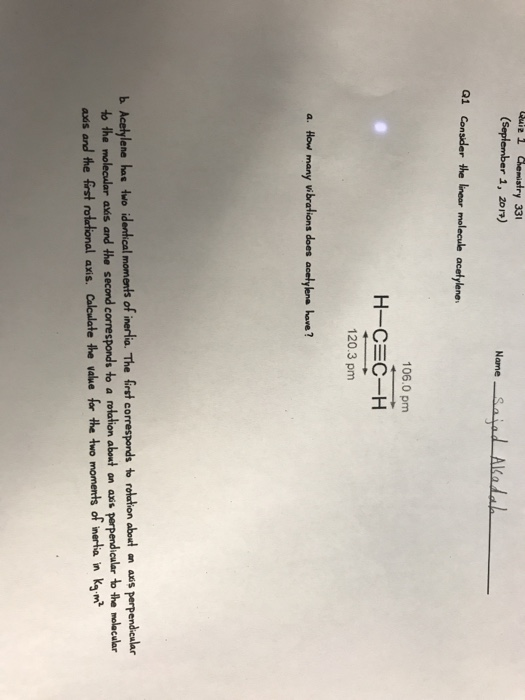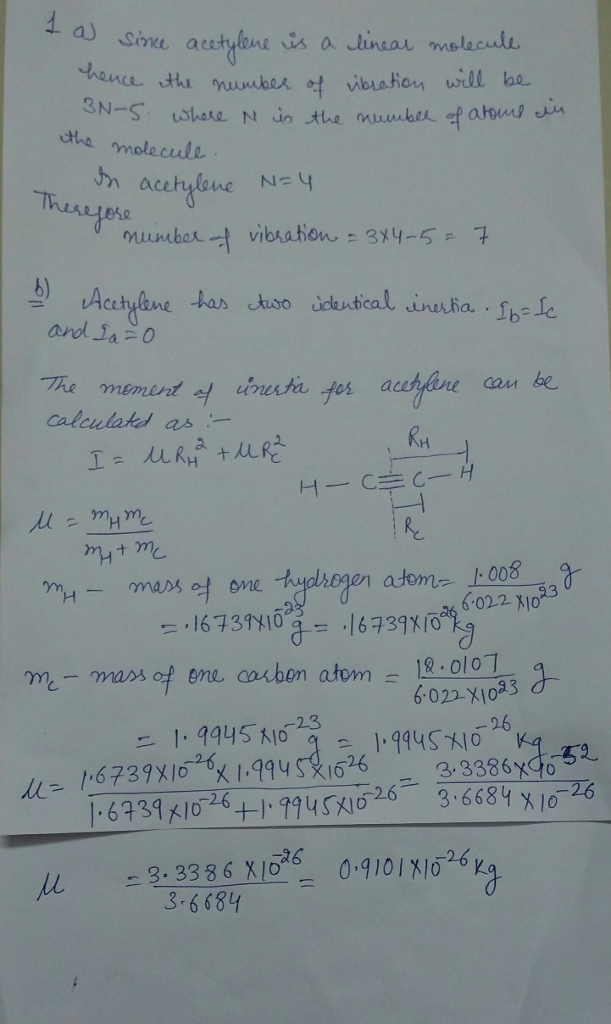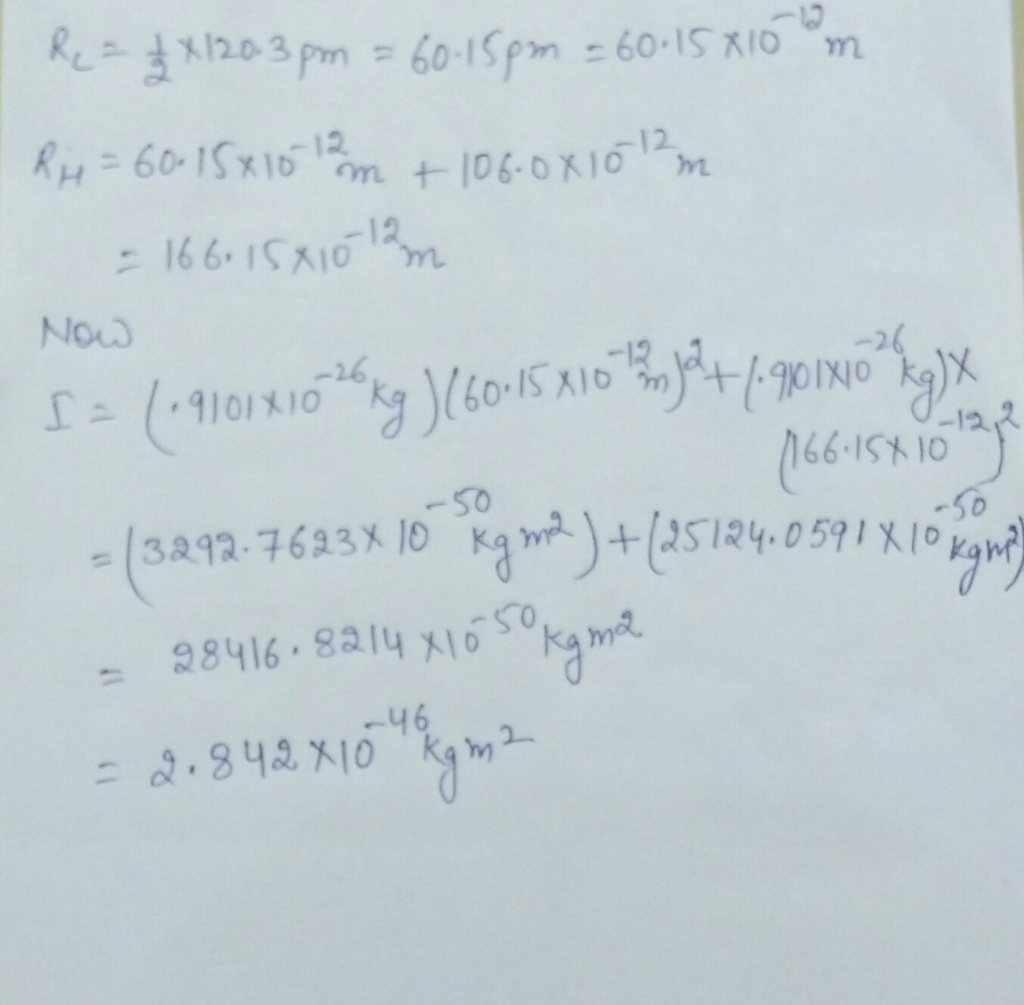# Question & Answer: Consider the linear molecule acetylene. a. How many vibrations does acetylene have? b……Consider the linear molecule acetylene. a. How many vibrations does acetylene have? b. Acetylene has two identical moments of inertia. The first corresponds to rotation about an axis perpendicular to the molecular axis and the second corresponds to a rotation about an axis perpendicular to the molecular axis and the first rotational axis. Calculate the value for the two moments of inertia in kg middot m^2